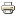# The degree measure as utility function over positions in networksWorking paper
Author/s:
René van den Brink, Agnieszka Rusinowska
Issue number:
2017.35
Series:
CES Documents de travail
Publisher:
Centre d'Economie de la Sorbonne
Year:
2017
In this paper, we connect the social network theory on centrality measures to the economic theory of preferences and utility. Using the fact that networks form a special class of cooperative TU-games, we provide a foundation for the degree measure as a von Neumann-Morgenstern expected utility function reflecting preferences over being in different positions in different networks. The famous degree measure assigns to every position in a weighted network the sum of the weights of all links with its neighbours. A crucial property of a preference relation over network positions is neutrality to ordinary risk. If an expected utility function over network positions satisfies this property and some regularity properties, then it must be represented by a utility function that is a multiple of the degree centrality measure. We show this in three steps. First, we characterize the degree measure as a centrality measure for weighted networks using four natural axioms. Second, we relate these network centrality axioms to properties of preference relations over positions in networks. Third, we show that the expected utility function is equal to a multiple of the degree measure if and only if it represents a regular preference relation that is neutral to ordinary risk. Similarly, we characterize a class of affine combinations of the outdegree and indegree measure in weighted directed networks and deliver its interpretation as a von Neumann-Morgenstern expected utility function.
Developed by Paolo Gittoi